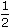# Aptitude - Stocks and Shares - Discussion

Discussion Forum : Stocks and Shares - General Questions (Q.No. 2)
2.
A man bought 20 shares of Rs. 50 at 5 discount, the rate of dividend being 13. The rate of interest obtained is:
 12 1 % 2
 13 1 % 2
15%
 16 2 % 3
Explanation:

Investment = Rs. [20 x (50 - 5)] = Rs. 900.

Face value = Rs. (50 x 20) = Rs. 1000.

 Dividend = Rs.27 x 1000= Rs. 135. 2 100

 Interest obtained =135 x 100% = 15% 900

Discussion:
27 comments Page 1 of 3.

Anomie said:   1 year ago
Thanks for explaining. @Krishna.

Yeshi Samdup said:   2 years ago
Great one, Thanks @Krishna.

Mrityunjay said:   2 years ago
27/2 rupees on 100 as dividend refers.
In question's case, it is 27/2 rupees on investing 90rs,
So simply find out 27/2 is what percent of 90.
(27/2)*100 /90 = 15.
That is the profit.

Face value = Rs. 50, Dividend = Rs. 27/2.
Market value = After Rs. 5 discount = 50 - 5 = Rs. 45,
Interest rate = Dividend / Market value * Face value.
= 27/2 / 45 * 50,
= 15,
= 15 %.
(1)

Saru said:   3 years ago
Yes, Understand, Thank You @Krishna.

Nikhitha said:   5 years ago
Thank you @Krishna.

Habib Rahman said:   6 years ago
20 * 50 = 1000, 20 * 45 = 900, 27 * 1000/2 * 100 = 135, 135 * 100/900 = 15%.

Komal said:   6 years ago
Simply 50 - 5 = 45.

20 * 45=900.
100 * 13.5=135.
Face value: 20 * 50 = 1000,
135 ÷ 900 * 100 = 15%.
(1)

Poonam said:   7 years ago
Actually, I have of listened a lecture on stock and shares. There it was said that total dividend is equal to a number of stocks multiply by dividend percentage. So my answer was 30 percent. I am really confused. Please explain.

Leo said:   7 years ago
Guys! I want to ask a question, because I don't get it how it could be solved!!

How many Rs. 25 shares at Rs 3 discount can be bought for Rs 2222, brokerage being 1%?
(1)